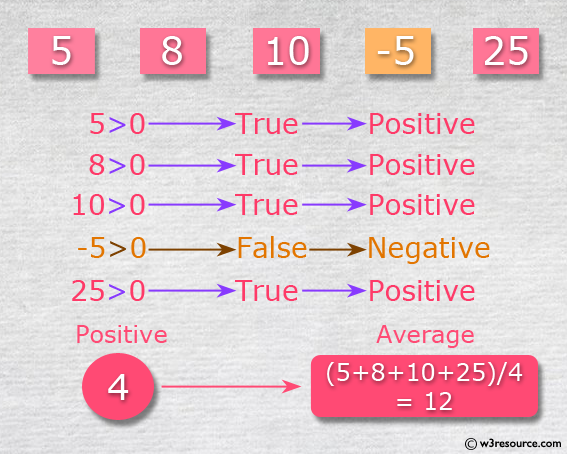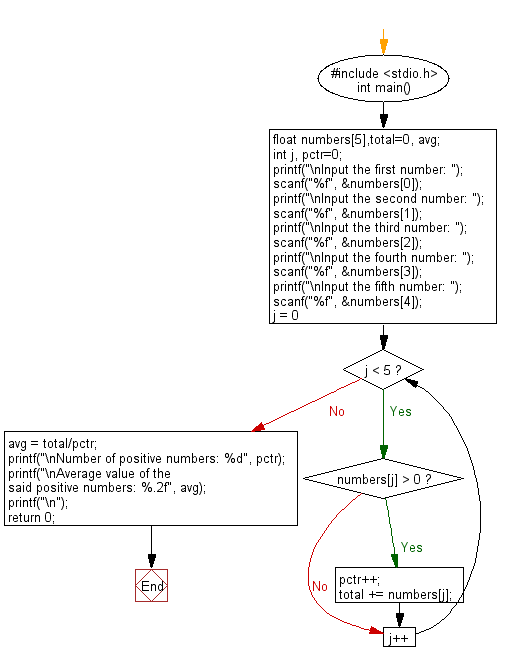﻿ C Program: Prints the average of all positive values# C Exercises: Counts the number of positive numbers and print the average of all positive values

## C Basic Declarations and Expressions: Exercise-28 with Solution

Write a C program that read 5 numbers and counts the number of positive numbers and print the average of all positive values.

Pictorial Presentation:C Code:

``````#include <stdio.h>
int main() {
float numbers,total=0, avg;
int j, pctr=0;
printf("\nInput the first number: ");
scanf("%f", &numbers);
printf("\nInput the second number: ");
scanf("%f", &numbers);
printf("\nInput the third number: ");
scanf("%f", &numbers);
printf("\nInput the fourth number: ");
scanf("%f", &numbers);
printf("\nInput the fifth number: ");
scanf("%f", &numbers);
for(j = 0; j < 5; j++) {
if(numbers[j] > 0)
{
pctr++;
total += numbers[j];
}
}
avg = total/pctr;
printf("\nNumber of positive numbers: %d", pctr);
printf("\nAverage value of the said positive numbers: %.2f", avg);
printf("\n");
return 0;
}
``````

Sample Output:

```Input the first number: 5

Input the second number: 8

Input the third number: 10

Input the fourth number: -5

Input the fifth number: 25

Number of positive numbers: 4
Average value of the said positive numbers: 12.00
```

Flowchart:C Programming Code Editor:

What is the difficulty level of this exercise?

Test your Programming skills with w3resource's quiz.

﻿

## C Programming: Tips of the Day

Is there a way to specify how many characters of a string to print out using printf()?

The basic way is:

`printf ("Here are the first 8 chars: %.8s\n", "A string that is more than 8 chars");`

The other, often more useful, way is:

`printf ("Here are the first %d chars: %.*s\n", 8, 8, "A string that is more than 8 chars");`

Here, you specify the length as an int argument to printf(), which treats the '*' in the format as a request to get the length from an argument.

You can also use the notation:

```printf ("Here are the first 8 chars: %*.*s\n",
8, 8, "A string that is more than 8 chars");```

This is also analogous to the "%8.8s" notation, but again allows you to specify the minimum and maximum lengths at runtime - more realistically in a scenario like:

`printf("Data: %*.*s Other info: %d\n", minlen, maxlen, string, info);`

The POSIX specification for printf() defines these mechanism

Ref : https://bit.ly/3u32GyO